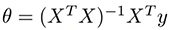2 views

what is the benefit of using Gradient Descent in the linear regression space? looks like we can solve the problem (finding theta0-n that minimum the cost func) with the analytical method so why we still want to use gradient descent to do the same thing? Thanks

by (33.1k points)

If you want to solve this problem by using only linear regression then you need to use normal equations for solving the cost function analytically.

Equation:In the above equation, X is your matrix of input observations and y is your output vector. The problem with this operation is the time complexity of calculating the inverse of an nxn matrix which is O(n^3) and as n increases. This process is computationally expensive and time-consuming.

If you use Gradient Descent, then it would be a more efficient way to work on a large dataset. That's why we use optimization algorithms for faster and accurate computation.

I hope this solution will clear your doubts.